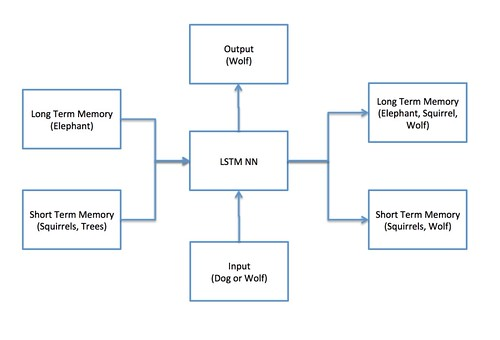Inner working of an AI that mimics human memory

In the last blog, I gave an overview of LSTMs (long short term memory) in AI that mimics human memory. I will use this blog to go 2 layers below to draw the building blocks of this technology.As a reminder, at a 50k level, the building block looks like the image on the left. There is long and short term memory on the left –> some input comes in –> new long and short term memory is output on the right. Plus, an output that determines what the input is.

Let’s open the box called the LSTM NN (neural network). This block is composed of four blocks or gates:

• The Forget Gate
• The Learn Gate
• The Remember Gate
• The Use Gate

The intuitive understanding of the gates is as follows: When some new input comes in, the system determines what from the long term memory should be forgotten to make space for the new stuff coming in; this is done by the forget gate. Then, the learn gate is used to determine what should be learnt and dropped from the short term memory.

The processed output from these gates is fed to the remember gate which then updates the long term memory; in other words a new long term memory is formed based on the updated short term and the long term memory. Finally, the use gate kicks in and produces a new short term memory and an output.Going a level deeper:

Learn Gate

The learn gate is broken in two phases: Combine –> Ignore.

• Combine: In the combine step, the system takes in the short term memory and the input and combines them together. In the example, the output will be Squirrels, Trees and Dog/Wolf (we don’t know yet — see previous blog for context)
• Ignore: In the second phase, information that isn’t pertinent will be dropped. In the example, the information about trees is dropped because the show was about wild animals.

Forget Gate

The forget gate decides what to keep from the long term memory. In the example, the show is about wild animals but there was input about wild flora, so the forget gate decides it is going to drop information about the flora.

Remember Gate

This gate is very simple. It adds output from the Learn gate and Forget gate to form the new long term memory. In the example, the output will be squirrel, dog/wolf and elephants.

Use Gate

This gate combines the input from the learn gate

Math behind the various gates for mathematically inclined

Learn Gate

Combine phase (output = Nt)

Take the STM from time t-1, take the current event Et and pass them through a tanh function.

Nt = tanh (STM_t-1, Et)

Mathematically Nt = tanh (Wn [STM_t-1, Et] + bn) where Wn and bn are weight and bias vectors.

Then, the output from the combine phase is multiplied by another vectory called i_t that is the ignore factor from the Ignore phase.

Ignore phase (output = i_t)

We create a new neural network that takes the input and STM and apply the sigmoid function on them.

i_t = sigmoid ( Wi [STM_t-1, Et] + bi)

Thus, the output from the Learn gate is:

tanh (Wn [STM_t-1, Et] + bn) * sigmoid ( Wi [STM_t-1, Et] + bi)

Forget Gate

The forget output is calculated by multiplying the long term memory with a forget factor (ft).

The forget factor is calculated using the short term memory and the input.

ft = sigmoid( Wf [STM_t-1, Et] + bf]

Forget output = LTM_t-1 * ft

Remember Gate

The remember gate takes output from the Forget and Learn gates and adds them together.

LTMt = LTM_t-1 * ft + Nt * i_t

Use Gate

The use gate applies a tanh function on the output of the forget gate and multiplies it to a sigmoid of the input and the event.

STMt = tanh (Wu [STM_t-1, Et] + bu) * sigmoid ( Wv [STM_t-1, Et] + bv)

Summary

The four gates mimic a point in time memory system. To truly envision this, think of a lattice of connected cells separated in time. Thus, the memory system will continually evolve and learn over a period of time.

(credit: the math and the example are coming in from the Udacity deep learning coursework)